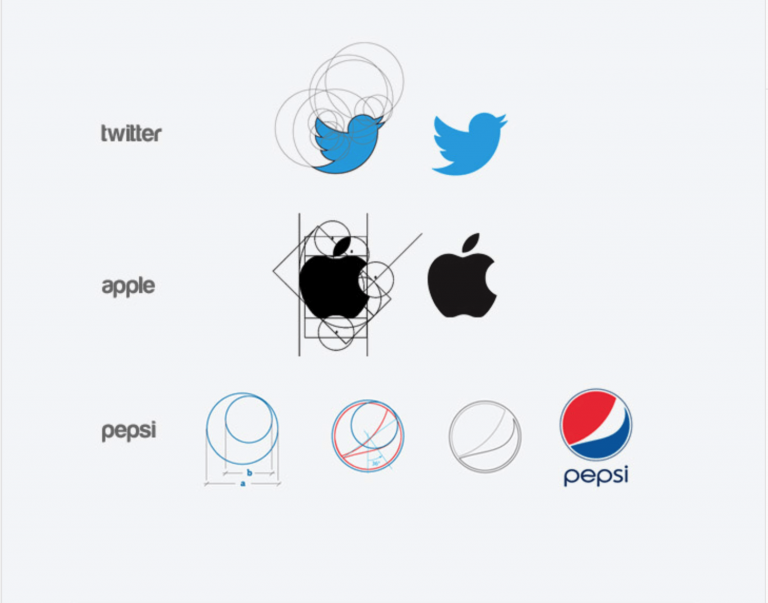# A guide to the Golden Ratio for designers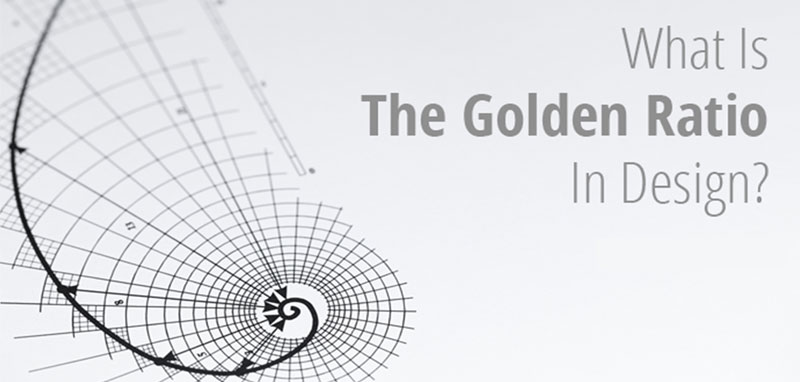### What is the Golden Ratio?

Source: Copied from invisionapp. Original link can be

Also known as the Golden Section, Golden Mean, Divine Proportion, or the Greek letter Phi, the Golden Ratio is a special number that approximately equals 1.618. The ratio itself comes from the Fibonacci sequence, a naturally occurring sequence of numbers that can be found everywhere, from the number of leaves on a tree to the shape of a seashell.

The Fibonacci sequence is the sum of the two numbers before it. It goes: 0, 1,1, 2, 3, 5, 8, 13, 21, and so on, to infinity. From this pattern, the Greeks developed the Golden Ratio to better express the difference between any two numbers in the sequence.

How does this relate to design? You can find the Golden Ratio when you divide a line into two parts and the longer part (a) divided by the smaller part (b) is equal to the sum of (a) + (b) divided by (a), which both equal 1.618. This formula can help you when creating shapes, logos, layouts, and more.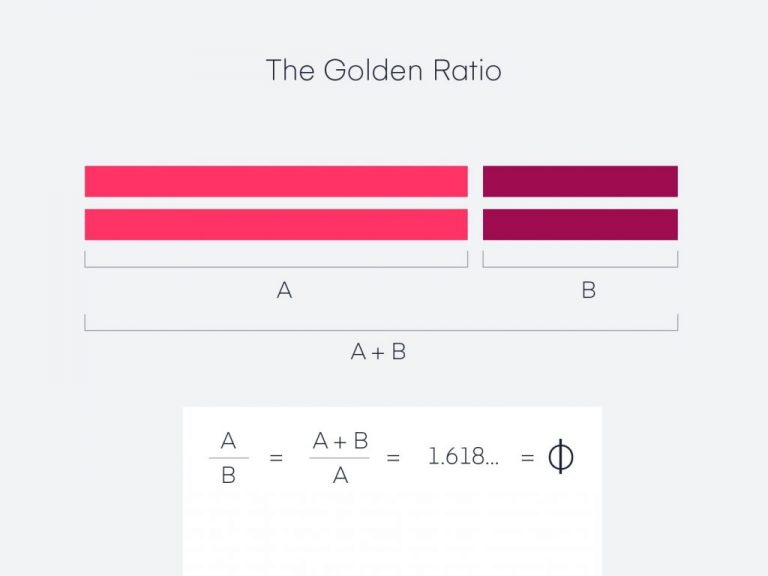You can also take this idea and create a golden rectangle. Take a square and multiple one side by 1.618 to get a new shape: a rectangle with harmonious proportions.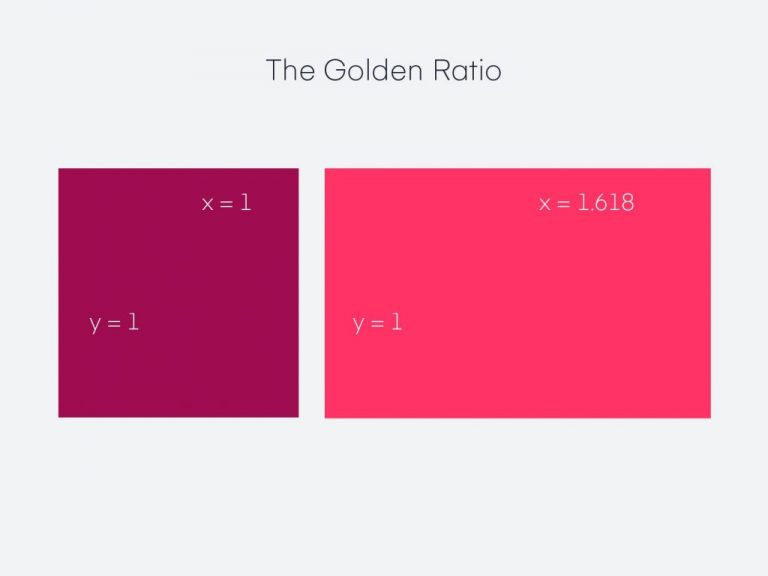If you lay the square over the rectangle, the relationship between the two shapes will give you the Golden Ratio.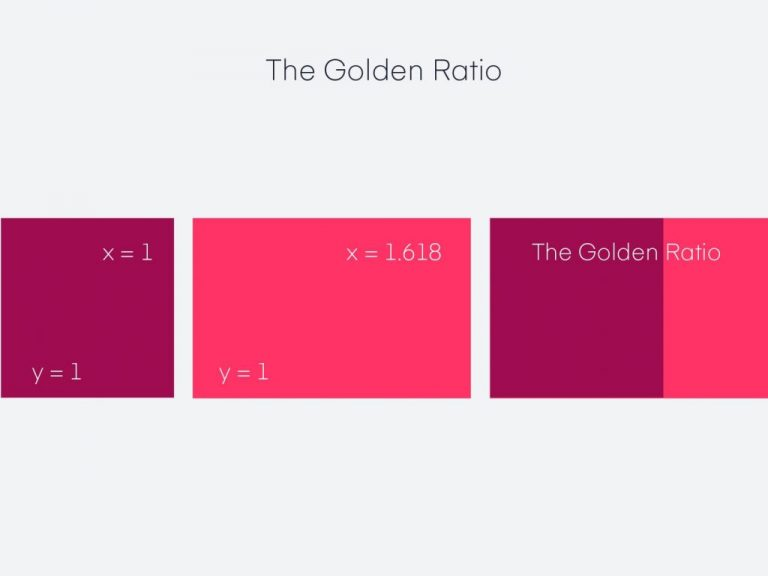If you keep applying the Golden Ratio formula to the new rectangle on the far right, you will end up with an image made up of increasingly smaller squares.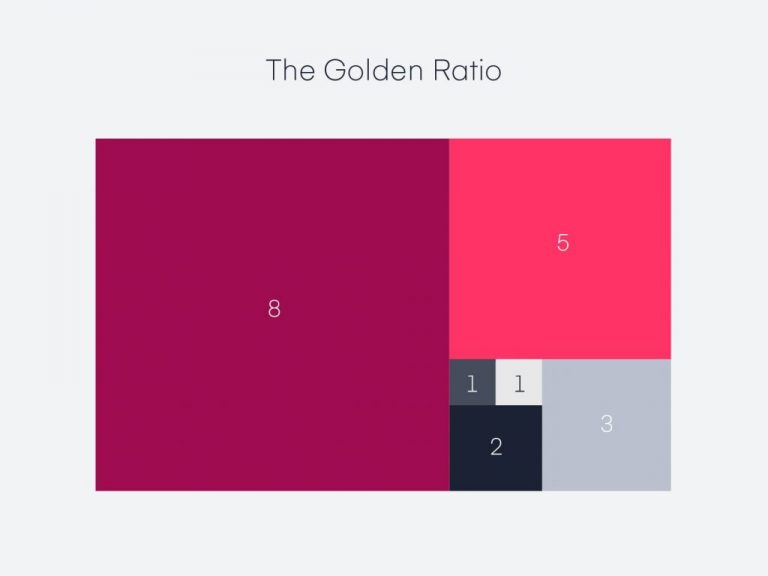If you draw a spiral over each square, starting in one corner and ending in the opposite one, you’ll create the first curve of the Fibonacci sequence (also known as the Golden Spiral).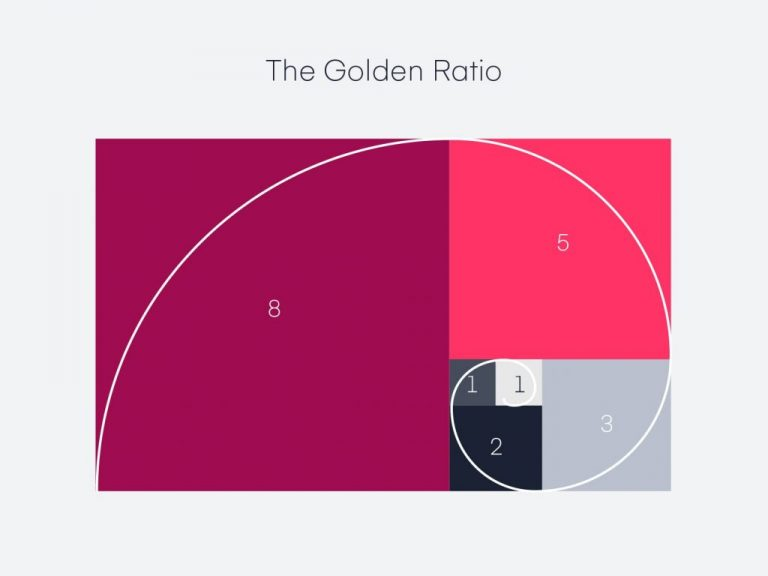## How to use the Golden Ratio in design

Now that the math lesson is over, how can you apply this knowledge to the work you do on a daily basis?

Here are four ways to use the Golden Ratio in design:

### 1. Typography and defining hierarchy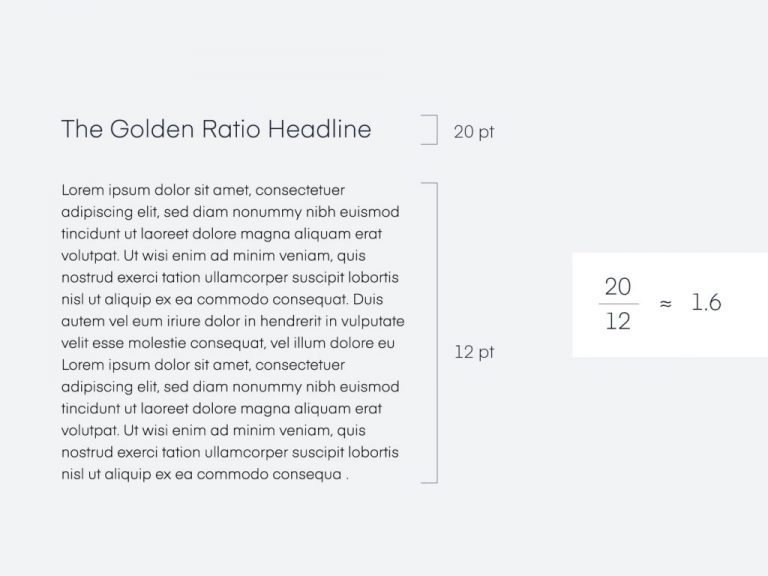The Golden Ratio can help you figure out what size font you should use for headers and body copy on a website, landing page, blog post, or even print campaign.

Let’s say your body copy is 12px. If you multiply 12 by 1.618, you’ll get 19.416, meaning a header text size of 19px or 20px would follow the Golden Ratio and balance the 12px body font size.

If you want to figure out how big your body text size should be, you could do the opposite. If your header text is 25px, you can divide it by 1.618 to find the body text (15 or 16 px).

### 2. Cropping and resizing images

When cropping images, it’s easy to identify white space to cut out. But, how do you make sure the image is still balanced after you resize it? You can use the Golden Spiral as a guide for the image’s composition.

For example, if you overlay the Golden Spiral on an image, you can make sure that the focal point is in the middle of the spiral.

### 3. Layout

Leveraging the Golden Ratio can help you design a visually appealing UI that draws the user’s attention to what matters the most. For example, a page that highlights a wide block of content on the left with a narrower column on the right can follow the Golden Ratio’s proportions and help you decide where to put the most important content.

### 4. Logo development